Home | | Discrete Time Systems and Signal Processing | Z Tranform - Analysis of LTI System

# Z Tranform - Analysis of LTI System

For analysis of continuous time LTI system Laplace transform is used. And for analysis of discrete time LTI system z transform is used. Z

ANALYSIS OF LTI SYSTEM

Z TRANFORM

INTRODUCTION TO Z TRANSFORM

For analysis of continuous time LTI system Laplace transform is used. And for analysis of discrete time LTI system z transform is used. Z transform is mathematical tool used for conversion of time domain into frequency domain (z domain) and is a function of the complex valued variable Z. The z transform of a discrete time signal x(n) denoted by

X(z) and given asZ transform is an infinite power series because summation index varies from -∞ to ∞. But it is useful for values of z for which sum is finite. The values of z for which f (z) is finite and lie within the region called as “region of convergence (ROC).

1.        The DFT can be determined by evaluating z transform.

2.        Z transform is widely used for analysis and synthesis of digital filter.

3.        Z transform is used for linear filtering. z transform is also used for finding Linear convolution, cross-correlation and auto-correlations of sequences.

4.        In z transform user can characterize LTI system (stable/unstable, causal/anti-causal) and its response to various signals by placements of pole and zero plot.

1.        ROC is going to decide whether system is stable or unstable.

2.        ROC decides the type of sequences causal or anti-causal.

3.        ROC also decides finite or infinite duration sequences.

Z TRANSFORM PLOTFig show the plot of z transforms. The z transform has real and imaginary parts. Thus a plot of imaginary part versus real part is called complex z-plane. The radius of circle is 1 called as unit circle. This complex z plane is used to show ROC, poles and zeros. Complex variable z is also expressed in polar form as Z= re where r is radius of circle is given by |z| and ω is the frequency of the sequence in radians and given by ∟z.Q) Determine z transform of following signals. Also draw ROC. i) x(n)= {1,2,3,4,5}

ii) x(n)={1,2,3,4,5,0,7}

Q) Determine z transform and ROC for x(n) = (-1/3)n u(n) –(1/2)n u(-n-1). Q)

Determine z transform and ROC for x(n) = [ 3.(4n)–4(2n)] u(n).

Q) Determine z transform and ROC for x(n) = (1/2)n u(-n).

Q) Determine z transform and ROC for x(n) = (1/2)n {u(n) – u(n-10)}.

Q) Find linear convolution using z transform. X(n)={1,2,3} & h(n)={1,2}

PROPERTIES OF Z TRANSFORM (ZT)

1) Linearity

The linearity property states that if zz Transform of linear     combination of two or more signals is equal to the same linear combination of z transform of individual signals.

2) Time shifting

The Time shifting property states that if z x(n)Thus shifting the sequence circularly by „k  samples is equivalent to multiplying its z transform by z –k

3) Scaling in z domain

This property states that if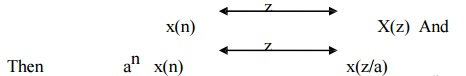Thus scaling in z transform is equivalent to multiplying by an in time domain.

4) Time reversal Property

The Time reversal property states that if zIt means that if the sequence is folded it is equivalent to replacing z by z-1 in z domain.

5) Differentiation in z domain

The Differentiation property states that if z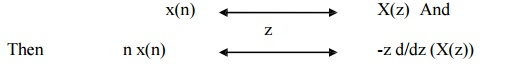6) Convolution Theorem

The Circular property states that if zConvolution of two sequences in time domain corresponds to multiplication of its Z transform sequence in frequency domain.

7) Correlation Property

The Correlation of two sequences states that if z8) Initial value Theorem

Initial value theorem states that if z9) Final value Theorem

Final value theorem states that if zRELATIONSHIP BETWEEN FOURIER TRANSFORM AND Z TRANSFORM.

There is a close relationship between Z transform and Fourier transform. If we replace the complex variable z by e –jω, then z transform is reduced to Fourier transform.

Z transform of sequence x(n) is given by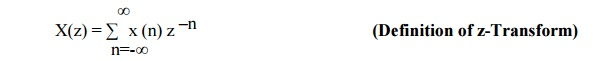Fourier transform of sequence x(n) is given byComplex variable z is expressed in polar form as Z= rejω where r= |z| and ω is ∟z. Thus we can be written as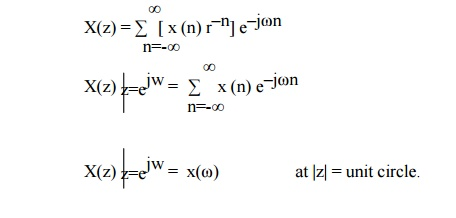Thus, X(z) can be interpreted as Fourier Transform of signal sequence (x(n) r–n). Here r–n grows with n if r<1 and decays with n if r>1. X(z) converges for |r|= 1. hence Fourier transform may be viewed as Z transform of the sequence evaluated on unit circle. Thus The relationship between DFT and Z transform is given byThe frequency ω=0 is along the positive Re(z) axis and the frequency ∏/2 is along the positive Im(z) axis. Frequency ∏ is along the negative Re(z) axis and 3∏/2 is along the negative Im(z) axis.INVERSE Z TRANSFORM (IZT)

The signal can be converted from time domain into z domain with the help of z transform (ZT). Similar way the signal can be converted from z domain to time domain with the help of inverse z transform(IZT). The inverse z transform can be obtained by using two different methods.

1) Partial fraction expansion Method (PFE) / Application of residue theorem

2) Power series expansion Method (PSE)

1. PARTIAL FRACTION EXPANSION METHOD

In this method X(z) is first expanded into sum of simple partial fraction.The above equation can be written in partial fraction expansion form and find the coefficient AK and take IZT.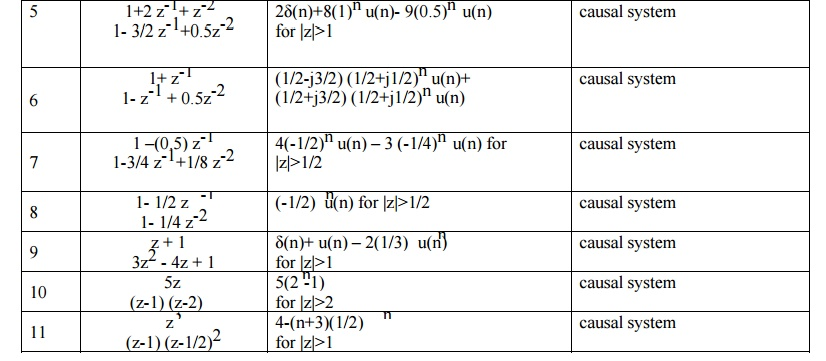2. RESIDUE THEOREM METHOD

In this method, first find G(z)= zn-1 X(Z) and find the residue of G(z) at various poles of X(z).3. POWER-SERIES EXPANSION METHOD

The z transform of a discrete time signal x(n) is given asExpanding the above terms we have

x(z) = …..+x(-2)Z2+ x(-1)Z+ x(0)+ x(1) Z-1 + x(2) Z2 +…..        (2)

This is the expansion of z transform in power series form. Thus sequence x(n) is given as

x(n) ={ ….. ,x(-2),x(-1),x(0),x(1),x(2),…………..}.

Power series can be obtained directly or by long division method.

SOLVE USING “POWER SERIES EXPANSION“ METHODRECURSIVE ALGORITHMThus

X(0) = a0/b0

X(1) = 1/b0 [ a1- x(0) b1]

X(2) = 1/b0 [ a1- x(1) b1 - x(0) b2] ……………

SOLVE USING “RECURSIVE ALGORITHM“ METHODExample 2:Find the magnitude and phase plot ofExample 5:Find the inverse Z Transform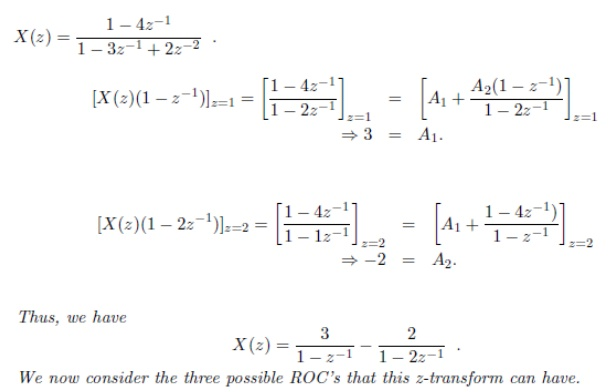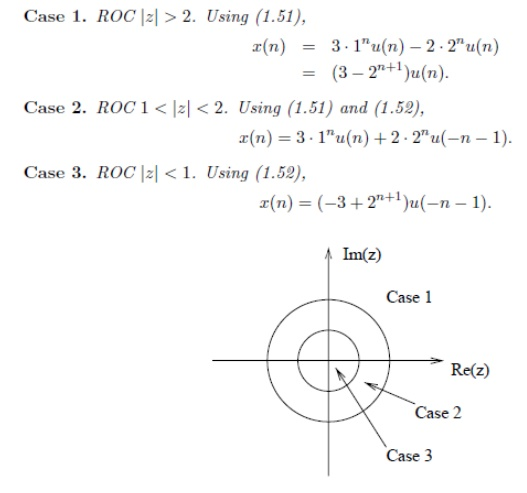POLE –ZERO PLOT

X(z) is a rational function, that is a ratio of two polynomials in z-1 or z.

The roots of the denominator or the value of z for which X(z) becomes infinite, defines locations of the poles. The roots of the numerator or the value of z forwhich X(z) becomes zero, defines locations of the zeros.

ROC dos not contain any poles of X(z). This is because x(z) becomes infinite at the locations of the poles. Only poles affect the causality and stability of the system.

CASUALTY CRITERIA FOR LSI SYSTEM

LSI system is causal if and only if the ROC the system function is exterior to

the circle. i. e |z| > r. This is the condition for causality of the LSI system in terms of z transform. (The condition for LSI system to be causal is h(n) = 0 ….. n<0 )

STABILITY CRITERIA FOR LSI SYSTEM

Bounded input x(n) produces bounded output y(n) in the LSI system only if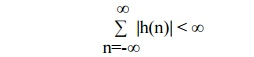With this condition satisfied, the system will be stable. The above equation states that the LSI system is stable if its unit sample response is absolutely summable. This is necessary and sufficient condition for the stability of LSI system.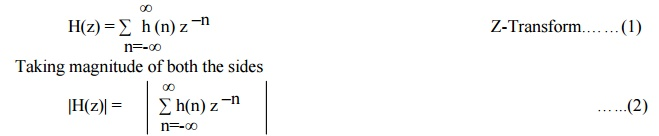Magnitudes of overall sum is less than the sum of magnitudes of individual sums.If H(z) is evaluated on the unit circle | z-n|=|z|=1.

Hence LSI system is stable if and only if the ROC the system function includes the unit circle. i.e r < 1. This is the condition for stability of the LSI system in terms of

z transform. Thus

For stable system |z| < 1

For unstable system |z| > 1

Marginally stable system |z| = 1

Poles inside unit circle gives stable system. Poles outside unit circle gives unstable system. Poles on unit circle give marginally stable system.

A causal and stable system must have a system function that converges for |z| > r < 1.

STANDARD INVERSE Z TRANSFORMSONE SIDED Z TRANSFORMProperties of one sided z transform are same as that of two sided z transform except shifting property.

1) Time delayExamples:

Q) Determine one sided z transform for following signals

1) x(n)={1,2,3,4,5}        2) x(n)={1,2,3,4,5}

SOLUTION OF DIFFERENTIAL EQUATION

One sided Z transform is very efficient tool for the solution of difference equations with nonzero initial condition. System function of LSI system can be obtained from its difference equation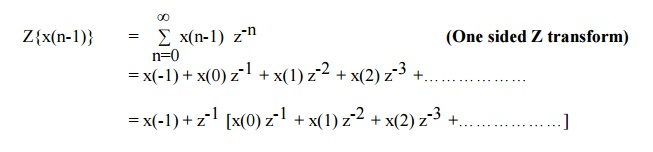Z{ x(n-1) } = z-1 X(z) + x(-1)

Z{ x(n-2) } = z-2 X(z) + z-1 x(-1) + x(-2)

Similarly

Z{ x(n+1) } = z X(z) - z x(0)

Z{ x(n+2) } = z2 X(z) - z1 x(0) + x(1)

Difference equations are used to find out the relation between input and output sequences. It is also used to relate system function H(z) and Z transform.

The transfer function H(ω) can be obtained from system function H(z) by putting z=e. Magnitude and phase response plot can be obtained by putting various values of ω.

First order Difference Equation

y(n) = x(n) + a y(n-1)

where          y(n) = Output Response of the recursive system x(n) =

Input signal

a= Scaling factor

y(n-1) = Unit delay to output. Now

we will start at n=0

n=0 y(0) = x(0) + a y(-1)                   ….(1)

n=1 y(1) = x(1) + a y(0)          ….(2)

= x(1) + a [ x(0) + a y(-1) ]

hence = a2 y(-1) + a x(0) + x(1)         ….(3)The first part (A) is response depending upon initial condition.

The second Part (B) is the response of the system to an input signal.

Zero state response (Forced response) : Consider initial condition are zero. (System is relaxed at time n=0) i.e y(-1) =0

Zero Input response (Natural response) : No input is forced as system is in non- relaxed initial condition.   i.e      y(-1) != 0

Total response is the sum of zero state response and zero input response.

Q) Determine zero input response for y(n) – 3y(n-1) – 4y(n-2)=0; (Initial Conditions are y(-1)=5 & y(-2)= 10) Answer: y(n)= 7 (-1)n + 48 (4)n

Q) A difference equation of the system is given below

Y(n)= 0.5 y(n-1) + x(n)

Determine   a)       System function

b) Pole zero plot

Unit sample response

A difference equation of the system is given below Y(n)= 0.7 y(n-1) – 0.12 y(n-2) + x(n-1) + x(n-2)

System Function b) Pole zero plot

Response of system to the input x(n) = nu(n)

Is the system stable? Comment on the result.

Q) A difference equation of the system is given below

Y(n)= 0.5 x(n) + 0.5 x(n-1)

Determine

a) System function

b) Pole zero plot

Unit sample response

Transfer function

Magnitude and phase plot

A difference equation of the system is given below a. Y(n)= 0.5 y(n-1) + x(n) + x(n-1)

Y(n)= x(n) + 3x(n-1) + 3x(n-2) + x(n-3)

System Function b) Pole zero plot

Unit sample response

Find values of y(n) for n=0,1,2,3,4,5 for x(n)= δ(n) for no initial condition.

Q) Solve second order difference equation

2x(n-2) – 3x(n-1) + x(n) = 3n-2 with x(-2)=-4/9 and x(-1)=-1/3.

Q) Solve second order difference equation x(n+2) + 3x(n+1) + 2x(n) with x(0)=0 and x(1)=1.

Study Material, Lecturing Notes, Assignment, Reference, Wiki description explanation, brief detail
Digital Signal Processing : Signals and System : Z Tranform - Analysis of LTI System |